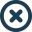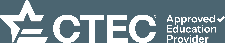Customer Service: 800-778-7436

Courses
Packages
CPE Topics
Who We Serve
Resources
Search# Microsoft® Excel® 2016: Useful Functions for CPAs (UF16)

• Credits 4
• Level Intermediate
• Field of Study Computer Software & Applications (Non-Technical) (4)

## Overview

This course guides you through creating formulas using Financial, Date & Time, Math & Trig, Statistical, Lookup & Reference, Database, Text and Logical functions.

## Major Topics:

• IRR, NPV, FV, PV, PMT, SLN, SYD, and DB Financial functions;
• NOW, TODAY, DATE, MONTH, DAY, YEAR, TIME, HOUR, MINUTE, SECOND, WEEKDAY, and NETWORKDAYS Date & Time functions;
• SUM, RAND, SQRT, and ROUND Math & Trig functions;
• AVERAGE, MAX, MIN, COUNT, MEDIAN, MODE.SNGL, and LARGE Statistical functions;
• VLOOKUP, HLOOKUP, and FORMULATEXT Lookup & Reference functions;
• DSUM, DCOUNT, DAVERAGE, DMIN, and DMAX Database functions;
• PROPER, SUBSTITUTE, and LOWER Text functions; and
• IF, AND, and IFNA Logical functions.

## Learning Objectives:

• Identify multiple ways to create a formula using one or more functions;
• Select Financial functions to calculate the internal rate of return, net present value, future value, present value, payment and depreciation;
• Select Date & Time functions to insert date and time components;
• Select Math & Trig functions to calculate the sum of a range of numbers; find a random number and the positive square root of a number; and round calculations to a specific number of places;
• Select Statistical functions to find the average, maximum, minimum, count, median and mode of a range of numbers plus the top five numbers;
• Select Lookup & Reference functions to find specific data and document worksheet formulas;
• Select specific criteria and Database functions to calculate the sum, average, maximum value, minimum value and number of cells containing numbers in a database field;
• Select Text functions to improve worksheet appearance; and
• Select Logical functions to perform calculations based on one or more tests.

## Who should take this course:

Experienced Excel 2016 users, or experienced Excel users switching from earlier versions.

Experience working with Excel 2016 in the Windows operating system environment

None

No

No

No